×

Data

# Understanding PREVIOUS_VALUE in TableauHow the heck does this function work?

You know what? I have been working with (and teaching) Tableau for over five years. Have a guess at when I finally understood the PREVIOUS_VALUE function … It was yesterday. And let’s get one thing straight right away: If you want to show the previous values of your field, don’t bother with this function because that’s the very thing it can’t do. (To show previous values, just use the LOOKUP function with an offset of -1. That does the trick.)

## Three Foundational Principles

So, what does this function do? There is no better way to learn something than to teach it, so here is a new blog that reduces a five-year learning path down to 15 minutes. Ready? Let’s get right into it and do the boring theory at the beginning (so we don’t have to bring it up later when it gets exciting). There are three primary lessons:

Lesson #1: Self-referring

PREVIOUS_VALUE is a self-referring function. When it was introduced long ago, it probably was the Tableau dream of everyone who fell in love with loops and recursive functions in other tools or languages.

Self-referring? Recursive? What are you talking about?

It sounds far more complicated than it is. And since a picture tells more than a thousand words, let’s look at these four tiny tables where the first two columns are calculated somehow in the third column. Next to that, we see the calculation. And while the blue ones on the left are just straight on calculations for each separate row, the red ones on the right are a little different: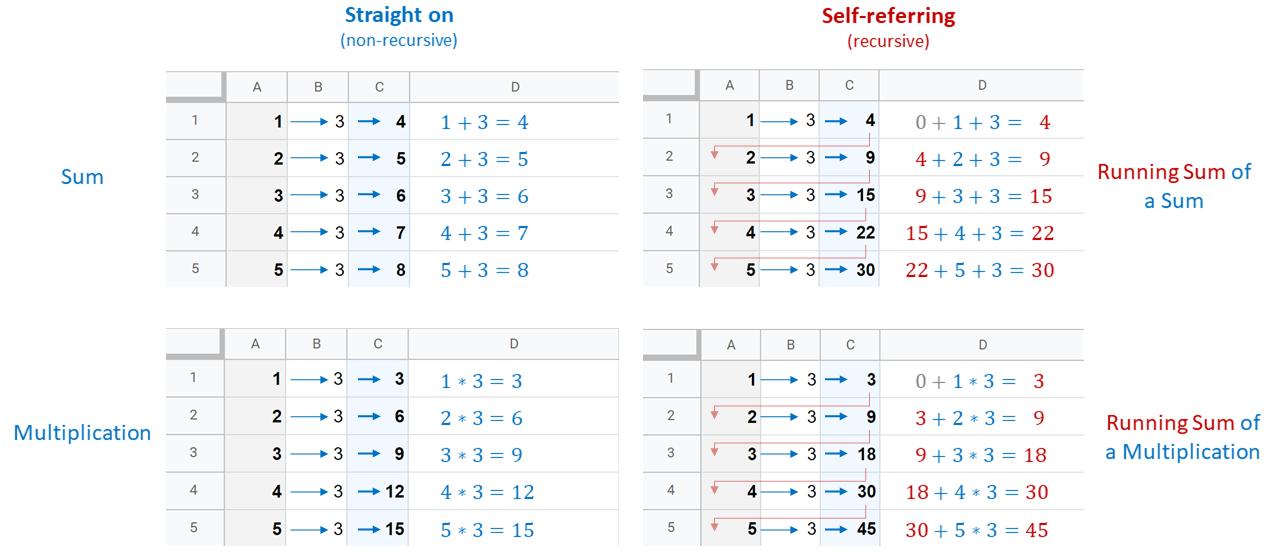On the right, we add the previous results for each row to the calculation of this row. This gives us a running sum and is already quite near to where we are going in Tableau. You may notice two grey zeros on the right charts. Keep those in mind!

Lesson #2: It’s a Table Calc

This is very important, as the PREVIOUS_VALUE function is neither an aggregation function nor a basic row-level function. When we replicate this function in Excel, we don’t have to think about it too much. In the lower-right chart on the image above, the cell C5 (for example) contains the following simple formula: =C4+A5*B5. In Excel, we just drag this formula up or down, and we get what we want without any complex functions.

But remember: Tableau is not a cell-based tool like Excel; it is table-based. Whatever we do with a cell in a column, we do with all cells in that column. Of course, calculations, filters, groups, sets and all the other beloved features in Tableau give us the freedom we want. Still, Tableau takes the whole column into account when calculating, not just a single cell. That means we have to consider the scope and direction of the table calculation when we implement it in our view.

That also means we have a problem when Tableau tries to calculate the very first row of our partition because that one does not have a previous value. This brings us to…

Lesson #3: Two Syntax Mysteries

The syntax of our function is fairly simple: PREVIOUS_VALUE(expression)

There are two things here: the first is something very important that this syntax is not telling us and the other is the expression.

What is it not telling?

The function PREVIOUS_VALUE alone is more or less pointless, unless you want to do some math (which is fine, of course). It works – strictly speaking – but there is no data value in it, quite literally. As we have already clarified, the function is self-referring, so when we want to give it something to do, we have to treat it like its own metric, rather than like a function, and use it in the very calculation we have in our mind, connecting it with the data we want to use it on via usual operators.

Erm … what?

Okay, okay, that was cryptic, but basically that just means: Put a measure into your field, so the function has something to work on. You want a running sum for the [Sales] metric? Let the function work with it:

`PREVIOUS_VALUE(0) + SUM([Sales])`

This translates to: “Dear function, please sum up [Sales] for this row and add what you calculated in the previous row.”

Okay. But I still don’t get it. What is this expression exactly? Why is there a 0 here and not the SUM([Sales])?

This expression drove me mad over so many years. Long story short: It is not an argument. It is not. When we want the previous value for the sum of Sales for any calculation, PREVIOUS_VALUE(SUM([Sales])) does not work at all, although it looks quite intuitive. Don’t get me wrong – the syntax is okay. There won’t be an error message. But we don’t get what we want. So, what is this expression?

It is the starting point of our PREVIOUS_VALUE function given that, for the very first row, there cannot be any previous value. That’s all the magic there is. Not more, not less. It does not calculate anything, it does not fetch a metric or dimension to work with, it just defines with what the function is going to start its journey. The initial value. Remember the grey zeros in our chart?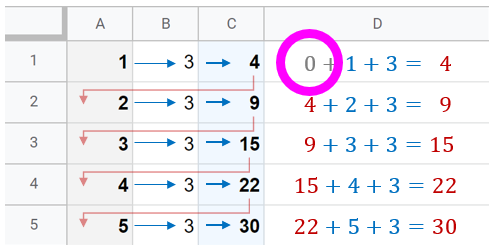In Excel or Google Spreadsheets, we get around this very easily. We just remove the reference to the impossible row before row 1. For example, for C2, the formula is =C1+A2+B2. But for C1 it becomes just =A1+B1 and we are fine.

In Tableau, we are table-based and cannot tinker with one specific cell. Fortunately, the expression is our way out: This zero is our starting point, at least when we build a running sum. When the function starts to work, it begins with 0 (zero).

In other words: “Dear function, please sum up [Sales] for this row and add what you calculated in the previous row. And when you’re just starting, please pretend you calculated a 0 in the previous row.”

## Time for Hands-on Practice

If everything feels a bit abstract right now, don’t worry, it is going to clear up. Just keep those three things we have learned in mind:

1. PREVIOUS_VALUE is self-referring.
2. It is a table calculation.
3. We have to give it work to do and the expression is just the starting point of that work.

The first step is to copy the following table and paste it into an empty Tableau sheet:

Year , Ones , Sequential , Currency (\$)
2009 , 1 , 1 , 100
2010 , 1 , 2 , 250
2011 , 1 , 3 , 1100
2012 , 1 , 4 , 55
2013 , 1 , 5 , 2010
2014 , 1 , 6 , 1500
2015 , 1 , 7 , 125
2016 , 1 , 8 , 246
2017 , 1 , 9 , 2123
2018 , 1 , 10 , 1234
2019 , 1 , 11 , 500
2020 , 1 , 12 , 500
2021 , 1 , 13 , 600
2022 , 1 , 14 , 860
2023 , 1 , 15 , 440

Next, right-click on the Sheet tab, and duplicate it as a Crosstab. Exclude the NULL values. Create a calculated field (“previous value”) that contains:

`PREVIOUS_VALUE(0) + SUM([Ones])`

Drag this new field into the Measure Values container: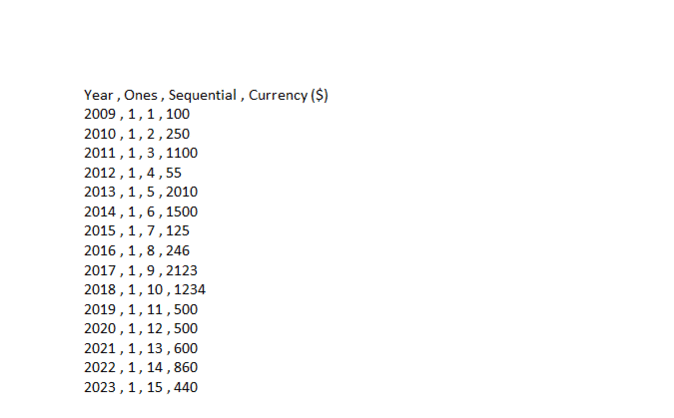Play a bit around with it, change the expression of the function, use it in another calculated field – there is no right or wrong, just learning.

And now, let’s take some questions!

I put a 200 into the function as its expression. That affects only the first value of the function, right?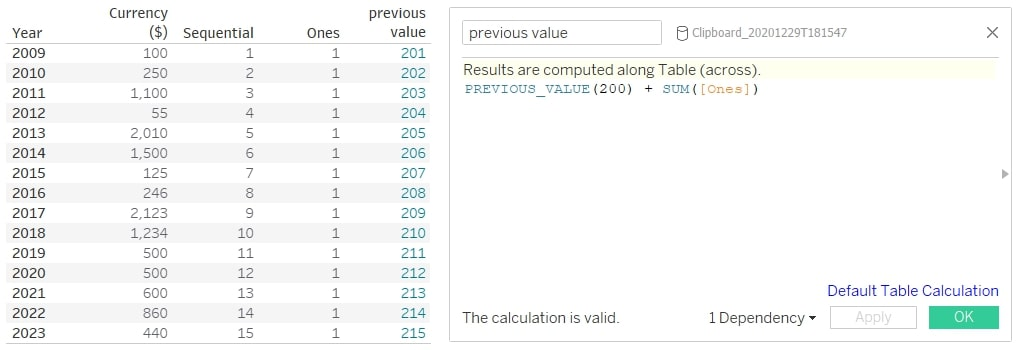The expression defines where PREVIOUS_VALUE starts its work, yes. In other words: Pretend there is a secret row in our table for the year 2008, and in there, this field has a value of 200. So, for our actual first row, PREVIOUS_VALUE adds 200 to 1 (that is coming from the field [Ones]).

What exactly happens when I put different measures into the calculation? Because it seems to work…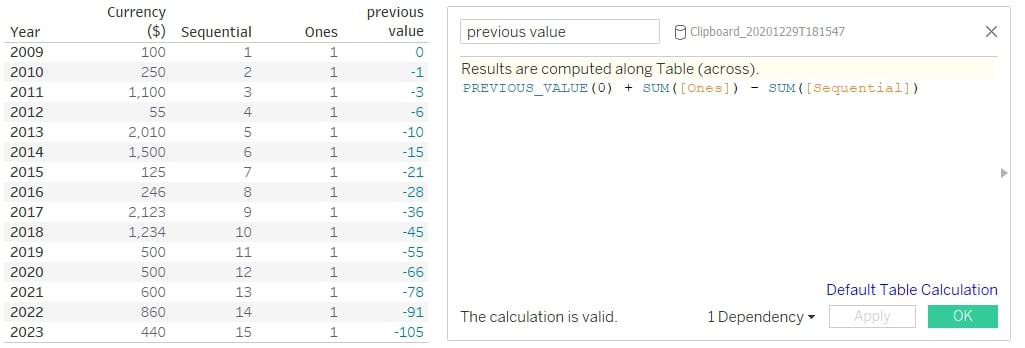It does! The calculation follows the usual rules of a calculated field. Within your field (that is a table calc), treat the PREVIOUS_VALUE function like another field in your mind. Basically, it does nothing else than fetch the previous result of this whole calculated field and calculate it however you define it.

In your case, it fetches the 0 from the expression, adds the 1 from the field [Ones] and subtracts the 1 from the field [Sequential] in your first row. For the year 2020, for example, it takes -55 from the year before, adds 1 and subtracts 12, arriving at -66.

I added a field as the expression – what is happening here?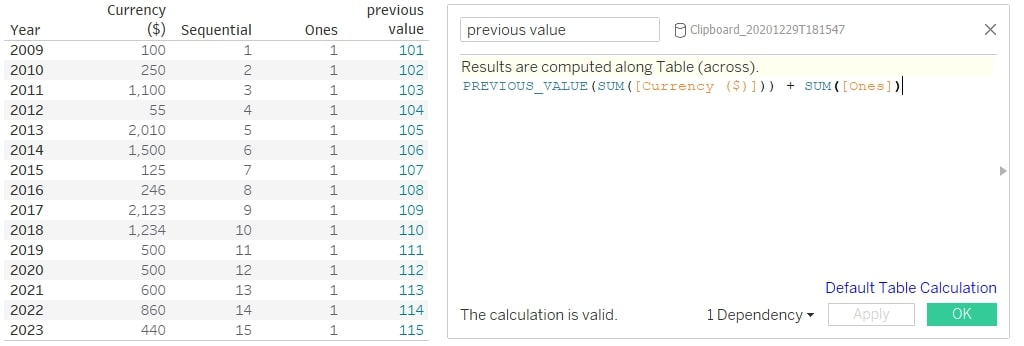When you put the SUM of [Currency (\$)] as expression into the function, you told PREVIOUS_VALUE to look into the first row of the currency field and use its value as a starting point. It takes the 100 from there, adds the 1 from [Ones] and gives 101 as a result for that first row. As it is a starting value, it cannot be several values. Only the very first value of the partition is the one that PREVIOUS_VALUE works with. Feels a bit arbitrary, but that’s how it is.

Does that mean I can even add a calculation in there as expression? Let me try…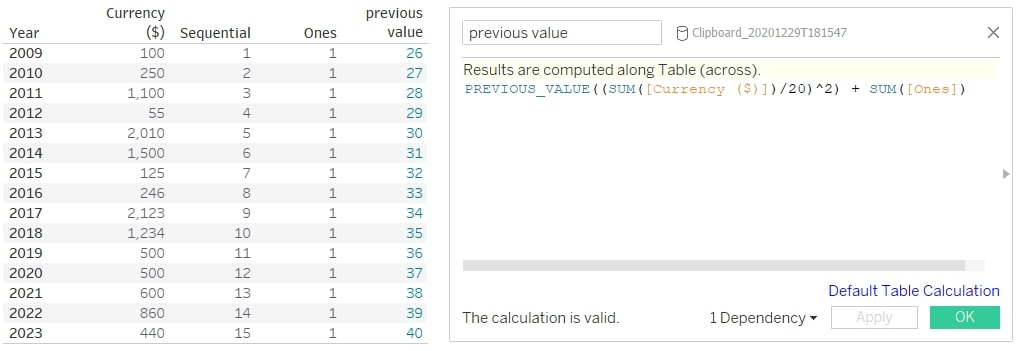Looks like it!

Yes! Perfect! As long as this calculation produces only one value, everything is fine.

Just out of curiosity: Why does PREVIOUS_VALUE not show the previous values in a column?

Good question! In some ways, the name of the function is a bit misleading. It works with all those previous values, but it does not show them. A more accurate name would be ALL_PREVIOUS_VALUES. The simplest way to show previous values would be a LOOKUP construct:

`LOOKUP(SUM([Currency (\$)]), -1)`

That’s also a table calculation, so be mindful of its computing direction.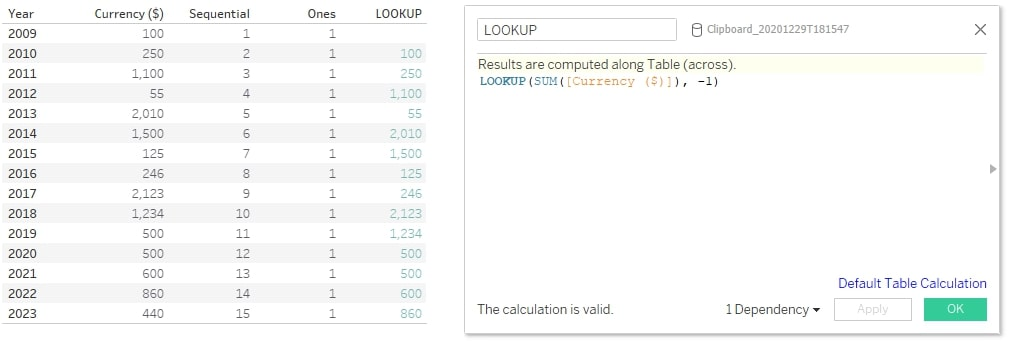The first row will return a NULL value here, as there’s no 2008 row the function can fetch a value from.

You said the PREVIOUS_VALUE function without any measure is pointless, unless I want to do math. Could you explain that?

The function in itself is self-referring. So, without any external value (like the currency measure before), it can only work with itself and everything math has to offer (which is a lot). In this respect, it does not differ from any other function. SUM(5 + 6) equals 11. That’s correct, but there’s no real data value here.

But while other functions need arguments they can calculate, PREVIOUS_VALUE is more or less its own argument, and putting a measure into the function (even a calculated one) does not do anything, except replicate this very starting value over all rows (here multiplied with 5). Have a look: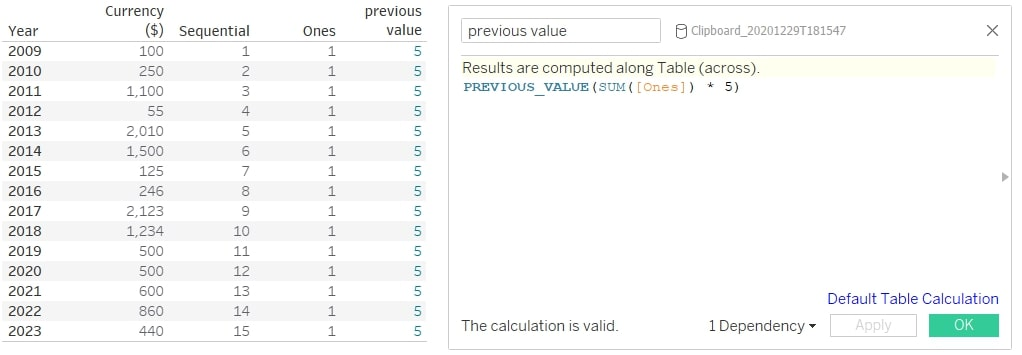Why does it do that?

Well, I didn’t order the function to do anything at all except tell it which starting value to use, meaning it always fetches the previous value, which was 5. But the moment we calculate literally anything with our PREVIOUS_VALUE function, the math gets quite powerful and very fast. For example: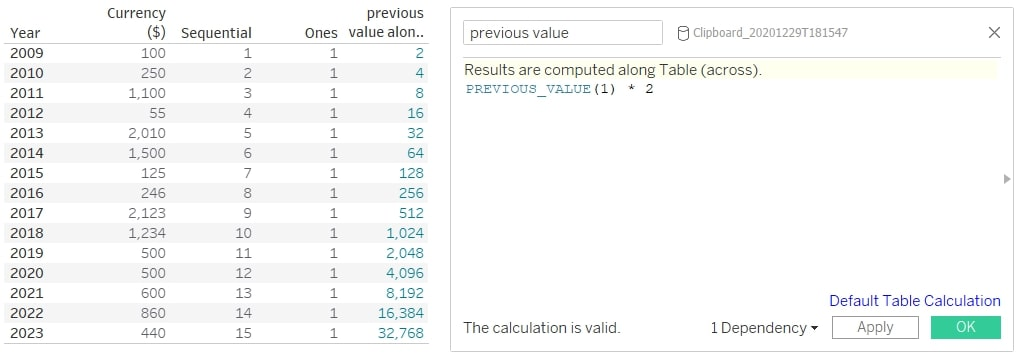Hm … when I try that, I get a 2 for each row.

Sounds like it is computing across instead of down. Check the direction of your table calculation. We have removed all other fields from the calculation, so Tableau may not know how to compute this. Make sure it’s set to Table (down) or Year. Then it should work.

Oh, so I can use products in there, too?

Yes. In terms of math, you can use whatever you like, as long as it plays along with the rules of math. Don’t forget that in spite of our lessons at the beginning, this function is fairly simple: It just takes the value from the previous row and does with it whatever we order it to do. Using products results in running products instead of running sums. That is powerful with or without a data field, as there is no RUNNING_PRODUCT function in Tableau. By the way: This also applies to differences and divisions.

Wait, that means I could calculate interests with that as well, right?

You mean, how much money you have after storing it in a vault at your bank since 2009? Easy!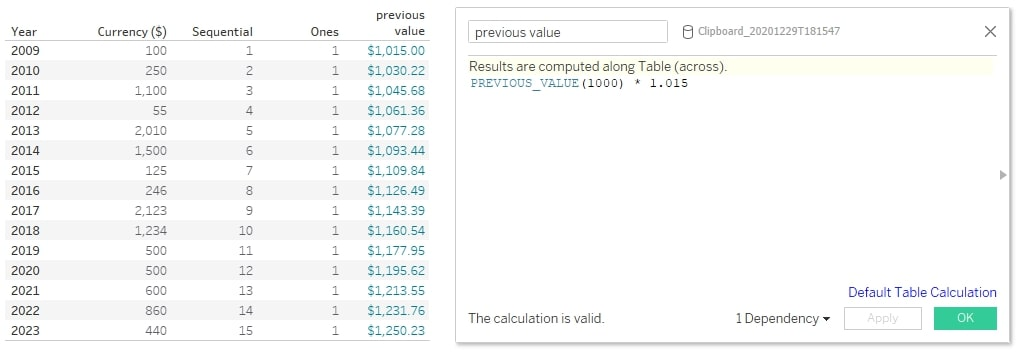Start with \$1000 in 2009, after negotiating an interest rate of 1.5%. Just play around with that simple construct.

That’s really cool. And I played around a bit. I think I want to see the interest numbers only, not the whole amount of money. But subtracting the \$1000 does not work. How do I do that?

Yes, here we also encounter one of the limits of PREVIOUS_VALUE. We cannot do this within our calculated field. You probably got something like this: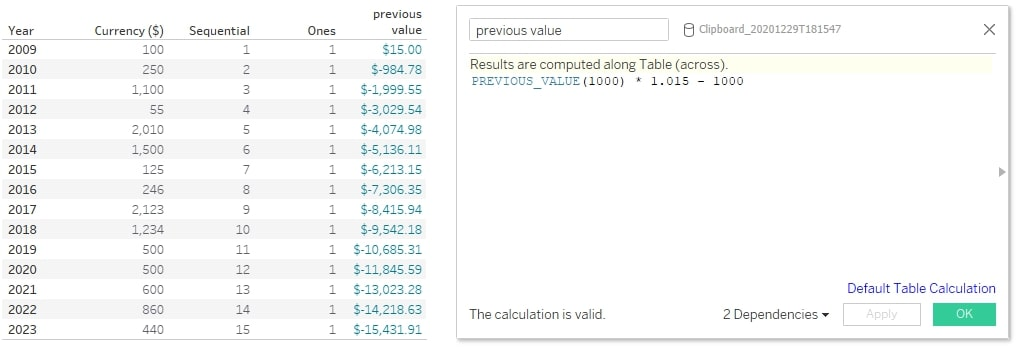This seems very wrong because the 1000 is subtracted in each row, again and again (that’s what we tell the field to do here). To get what we want, we would need to create another calculated field and just subtract the 1000 from there (like: [previous value] – 1000).

Does PREVIOUS_VALUE work with text? Sounds weird, I know…

A fair question. And the answer is yes. A value does not need to be a number at all; integers are just the default type for this function. However, with text, there are a lot of limitations, as all the cool math stuff is not possible. Nevertheless, the syntax does not change. Let’s summon a text field by converting our year numbers into characters in a new calculated field, like this:

`CHAR(INT(RIGHT(STR([Year]), 2)) + 88)`

Now, we can drag that new text field onto Rows, and we should have the letters a, b, c up to o next to the years.

Let’s bring up another calculated field for our PREVIOUS_VALUE function but this time with text: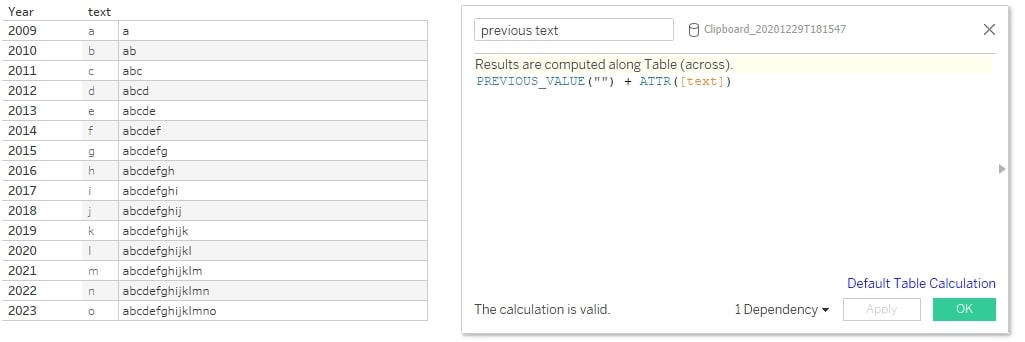It works! You may notice two differences: First, I did not use a number as the starting value (or expression) of our function but rather quotation marks, telling the function it works with texts. And second, I wrapped the text field into an ATTR function. That is necessary, as table calculations need aggregated values, and the attribute function emulates that for text fields.

Another example: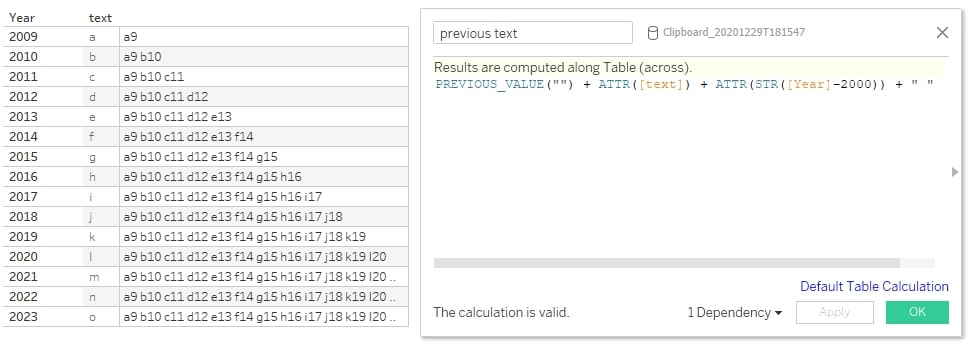And does it work with dates?

Try it out! Now you know how the function works, how to set the starting value and how to use everything in a calculated field. The rest is trial and error.

## That’s All for Today!

We covered the theory behind PREVIOUS_VALUE, learned three valuable lessons about it and did a lot of practice examples. If you have any more questions or are still struggling with this function, just ping me.

### The Latest

• ##### Tableau Hacks: The “Coat Hanger” Method

Why InterWorks? My name is Sebastian, I am an Analytics Lead in Germany and I just celebrated my three year anniversary at InterWorks. My job today is ...
Dynamic Zones in Tableau (or Hide and Show via Parameter) When Tableau version 2022.3 was released, expectations for new features were quite low in the Tableau community. There were a lot of ...

See more from this author →

InterWorks uses cookies to allow us to better understand how the site is used. By continuing to use this site, you consent to this policy. Review Policy OK

×

Interworks GmbH
Ratinger Straße 9
40213 Düsseldorf
Germany
Geschäftsführer: Mel Stephenson

Kontaktaufnahme: markus@interworks.eu
Telefon: +49 (0)211 5408 5301

Amtsgericht Düsseldorf HRB 79752
UstldNr: DE 313 353 072